# Power amplifier linearization technique with IQ imbalance and crosstalk compensation for broadband MIMO-OFDM transmitters

## Abstract

The design of predistortion techniques for broadband multiple input multiple output-OFDM (MIMO-OFDM) systems raises several implementation challenges. First, the large bandwidth of the OFDM signal requires the introduction of memory effects in the PD model. In addition, it is usual to consider an imbalanced in-phase and quadrature (IQ) modulator to translate the predistorted baseband signal to RF. Furthermore, the coupling effects, which occur when the MIMO paths are implemented in the same reduced size chipset, cannot be avoided in MIMO transceivers structures. This study proposes a MIMO-PD system that linearizes the power amplifier response and compensates nonlinear crosstalk and IQ imbalance effects for each branch of the multiantenna system. Efficient recursive algorithms are presented to estimate the complete MIMO-PD coefficients. The algorithms avoid the high computational complexity in previous solutions based on least squares estimation. The performance of the proposed MIMO-PD structure is validated by simulations using a two-transmitter antenna MIMO system. Error vector magnitude and adjacent channel power ratio are evaluated showing significant improvement compared with conventional MIMO-PD systems.

## 1. Introduction

Emerging broadband communication systems require high spectral efficiency and robustness against multipath channels. For this reason, OFDM has been adopted in the majority of modern wireless communication standards. Furthermore, multiantenna transceivers represent one of the most prominent techniques to enhance system capacity. Mobile WiMAX, LTE, Ultra Wide Band, and WLAN (IEEE 802.11n) allow the use of MIMO-OFDM (multiple input multiple output-OFDM) in their specifications. However, several factors should be considered to obtain the advantages promised by MIMO techniques. The high dynamic range of OFDM signals imposes the use of linear amplifiers (class A and class AB). The requirement of linear amplifiers, with a poor power duty, creates a problem accentuated by the use of multiple antennas. The high-data transmission rates, reached with the combination of OFDM and MIMO contrast with the loss of portability of the product, because of their elevated power consumption. Therefore, there is a trade-off between the high data rate obtained by employing MIMO techniques and the high power consumption of the OFDM system. Furthermore, when considering low-cost components, there are also several imperfections/impairments that degrade the system performance and need to be taken into account in the design of a compensation system.

Despite several advantages, OFDM is sensitive to distortions introduced at the RF front-end. Inexpensive OFDM transceivers employing direct conversion architectures (zero intermediate frequency) are seriously affected by front-end distortions, e.g., in-phase and quadrature (IQ) baseband imbalance and phase noise. In addition, OFDM transceivers are also intrinsically sensitive to power amplifier (PA) nonlinear distortion. Nonlinear PA creates spectral regrowth (out-of-band distortion) and in-band distortion that degrades the system's bit error rate (BER). The trade-off between power efficiency and linearity motivates the development of novel signal-processing techniques to reduce the nonlinear distortion.

Nonlinear distortion can be compensated either at the transmitter side or the receiver side. For the former case, the signal to be transmitted is modified before the PA, and among the well-known methods for this purpose are predistortion and PAPR reduction techniques. SISO predistortion methods aim to model the inverse of the PA nonlinear response. The predistorter (PD) is placed before the PA such that the cascade PD-PA produces a linearly amplified signal. The most simple PD structure is memoryless, where the current output depends only on the current input. In that case, the PD is described by a static nonlinear function often implemented with polynomial models . In broadband OFDM implementations, however, memory effects that appear in the PA response need to be considered in the PD design. Volterra, Wiener, Wiener-Hammerstein, and memory polynomial (MP) models are generally employed for these cases of PD design [2, 3].

Receiver-side compensation can be justified for uplink transmission moving the processing task to the base station where higher computational complexity is allowed. In this form, mobile terminals are kept simple and power efficient . It is worthwhile to mention that receiver-side compensation techniques need to deal with the estimation problems associated to the channel (i.e., memory effects and time-varying characteristics).

Imperfections in the IQ modulator represent another important issue in the design of a baseband PD. The predistorted baseband signal is up-converted to RF using an IQ modulator. Phase and amplitude imbalances of the modulator affect the estimation of the PD coefficients. This problem motivates the joint compensation of PA nonlinear response and the distortion introduced in the up-conversion process. The latter distortion is due to phase and amplitude imbalances of the local oscillator (LO) and mismatch in the cascade of digital-analog converters (DAC)-low-pass filters in the I and Q branches. The effects of IQ imbalance on predistortion techniques have been presented in . Joint PD and IQ compensation techniques have been previously considered in several studies for the SISO case .

On the other hand, the implementation of PD techniques in MIMO systems introduces specific problems mostly related to the crosstalk between the different branches. In multi-antenna systems, the crosstalk between the different MIMO channels affects severely the system performance. The crosstalk can be (a) after the transmitter antennas and (b) at the transmitter RF front-end (before PA). For case (a), the coupling process can be modeled as a linear crosstalk (it occurs after the transmitter PA), and is usually mitigated at the receiver side (in the channel equalization process) [12, 13]. In spite of this, the use of MIMO transceiver structures implemented on reduced size leads to the coupling case (b) between the branches at the front-end that cannot be avoided. This kind of crosstalk is amplified by a nonlinear device (amplifier) and is denoted as nonlinear crosstalk. The nonlinear crosstalk and the PA nonlinear response should be jointly compensated by a MIMO predistorter to get a reliable system performance.

A MIMO-PD for broadband systems including coupling effects has been reported in . In the cited article, it is shown that the use of multiple SISO PDs assuming N-independent paths (no crosstalk) gives poor results in terms of in-band distortion and adjacent channel power ratio (ACPR). On the other hand, the proposed PD coefficient estimation technique has high computational complexity, and IQ imbalance effects, and tracking the changes of PA characteristics in time due to temperature or bias point variations have not been considered in .

In this study we propose a MIMO-PD based on a parallel MP model that renders the following properties:

1. (a)

Compensation of nonlinear crosstalk and IQ imbalance effects: We propose a modified (parallel) MP  structure to linearize each broadband PA response and to compensate the (frequency-dependent) crosstalk and IQ imbalance effects.

2. (b)

Reduced complexity: The proposed MIMO-PD, based on the indirect learning scheme , uses efficient recursive least squares (RLS) and stochastic gradient (SG) algorithms (when compared with , for example) to estimate the MIMO-PD coefficients.

3. (c)

Robustness against measurement noise: Even when indirect-learning architectures are affected by measurement noise at the PA output (creating a bias in the estimated PD coefficients) , the performance of the MIMO-PD proposed is validated for practical scenarios (SNR > 30 dB), showing good results.

4. (d)

Tracking capability: Contrary to what is required in , the estimation of PD coefficients can be performed at the system initialization without a special training sequence. The proposed recursive implementation allows for updating the PD coefficients on-line to track the changes in the PA parameters.

The organization of the study is as follows. The MIMO-OFDM system model (including nonlinear PA, crosstalk effects and IQ imbalance imperfections) is described in Section 2. In Section 3, the proposed PD structure is introduced, and the specific efficient estimation algorithms are derived. Also, an analysis of complexity and implementation issues is included in this section. Section 4 presents simulation results to validate the performance of the new MIMO-PD scheme. In this section, the figures of merit employed to evaluate the proposed MIMO-PD are the error vector magnitude (EVM) and the ACPR. Finally, Section 5 concludes the article.

To simplify the notation, we develop the MIMO-PD for M = 2 transmit antennas. However, the proposed technique is not restricted to this case and can easily be generalized to M > 2 transmit antennas.

Throughout this article, we employ the following abbreviations. MIMO-PD denotes MIMO predistorter, and CPD represents conventional predistorter. MIMO-PD SG, MIMO-PD RLS, and MIMO-PD LS are employed to define the MIMO predistorters coefficients of which were obtained using stochastic gradient, recursive least squares, and least squares algorithms, respectively. The acronyms MP and MLP denote memory and memoryless polynomials.

## 2. System Model

The transmitter front-end considered uses direct-conversion architecture . This architecture presents several advantages when compared with the conventional super-heterodyne structure: small number of parts, low-mixing product spurs, few filters, and low current consumption .

Let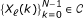be the modulated data symbols associated with carrier k to be transmitted by antenna = 1, 2,..., M. The time-domain OFDM symbols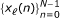are obtained via the inverse discrete Fourier transform: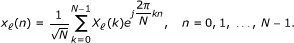(1)

The OFDM signal x (n) at the transmitter is separated into real and imaginary (IQ) digital baseband components, x i (n) and x q (n). The IQ components are filtered by the I and Q branches equivalent low-pass filters, h i (n) and h q (n), and converted to continuous-time baseband signals,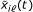and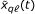.

The low-pass filters h i (n) and h q (n), which model the cascade of DAC and the analog low-pass filters, are represented as FIR filters of lengths L i and L q , respectively . Generically, the impulse responses h i (n) and h q (n) are different, creating an IQ frequency-dependent mismatch.

The IQ components at the low-pass filters output (continuous-time baseband signals) are directly modulated to RF, x rf (t), using two LO signals ideally in quadrature. However, in "real-life" implementations, LO signals present phase and amplitude imbalances in the I and Q branches. Amplitude- and phase imbalance parameters of the IQ-modulator associated to the branch are denoted as β and ϑ , respectively . Finally, the RF signal x rf (t) is amplified and transmitted through the channel, y (t). A block diagram of the transmitter front-end of the branch is illustrated in Figure 1.

Following the model described in , the equivalent discrete-time baseband signal after IQ modulator for each branch of the MIMO transmitter can be represented by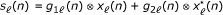(2)

where denotes convolution, g1(n) and g2(n) are equivalent filters with impulse response given by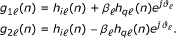(3)

Besides IQ imbalance, direct conversion transceivers suffer from DC offset because of LO leakage , the mixing of the LO signal with itself and noise from the mixers, filters, and DAC converters. The output of the IQ modulator including the DC offset term can be written as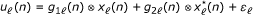(4)

where ε is the DC offset term due to imperfections at the up-converter. A block diagram of the described two-antenna MIMO-OFDM transmitter front-end (equivalent baseband model) is illustrated in Figure 2.

The IQ imbalance model given by (2) is composed by two branches and motivates the parallel structure of our MIMO-PD as presented in the next section.

MIMO transceiver RF front-end requires a careful design to isolate the different branches. Nevertheless, when considering reduced-size implementation (chipset), the coupling between the MIMO branches cannot be fully eliminated. To model this kind of crosstalk which is assumed frequency dependent, we consider the output of the PAs, written as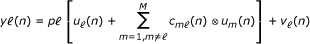(5)

where p [·] is the PA response of each branch, u m (n) is the output of the IQ modulator of the m path of the transceiver, and c m (n) is the filter representing the crosstalk with impulse response,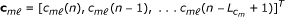and modeling the coupling between path m to path . The measurement noise at the output of each PA is denoted by v (n). Equation 5 allows inferring that to obtain a distortion-free signal x (n), the PD should be able to invert the PA response p [·], remove the undesired coupled signal, and mitigate the effects of the IQ imbalance.

## 3. MIMO Predistorter

Owing to the effects of crosstalk and IQ imbalance, the MIMO transmitter to be linearized follows a characteristic that can be described by a parallel nonlinear model. We consider, for the derivation, the linearization of one MIMO path. The PD coefficients are estimated using an indirect learning structure . In this methodology, the MIMO-PD parameters are estimated and copied to the predistorter avoiding the inverse model estimation required by direct learning techniques. However, despite several advantages, the indirect learning structure is affected by measurement noise at the PA output [9, 15]. Measurement noise creates a bias in the estimated model, which increases with the model order. The effects of the measurement noise on the proposed technique are discussed and evaluated following a specific application in Section 4.

The proposed identification structure requires a feedback path where the RF signal at the output of the PA is down-converted and translated to baseband. The components of the down-converter, filters, DAC, and mixer need to be carefully designed in order to minimize its harmful effects over the performance of the identification technique. In this approach of this study, an ideal feedback path is considered. It is assumed that the demodulation is implemented digitally minimizing the demodulation errors. A feedback path without IQ demodulator imbalance and nonlinear effects was also considered in previous publications . In [5, 11], errors in the feedback loop and techniques to remove its harmful effects are addressed. However, only frequency-independent imbalances are considered.

### A. MIMO predistorter structure

Even when other alternatives are possible, the proposed MIMO predistorter is based on the MP model . That model has been employed in predistortion techniques showing a very good performance . The main characteristics of the MP model, which we exploit regarding real-time applications are its modularity and simplicity.

Furthermore, alternative modeling of the static part of the MP can also be considered. Orthogonal polynomials alleviate the ill-conditioned problems associated with the conventional polynomial models . Generalized MP proposed by Morgan  should also be an interesting option with improved stability at a reasonable increase of the implementation complexity, but its use is not discussed here.

To include all the impairments, i.e., the nonlinear distortion and memory effects due to the PA, crosstalk coupling due to the MIMO structure, and the IQ imbalance distortion, we propose for each PA (of the M-antenna MIMO system) a (2 + 2(M - 1)) × 1 MISO PD. Each branch of the MISO PD is formed by a MP . There are two branches to model the own PA nonlinear distortion (associated to the IQ components of the IQ imbalance characterization) and 2(M - 1) to model the crosstalk associated to the other PAs.

The proposed MISO PD structure, for the case M = 2, is depicted in Figure 3. Each block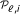denotes the MP associated to the branch i of antenna . Based on the M = 2 case, the (4 × 1) PD output associated to antenna can be written as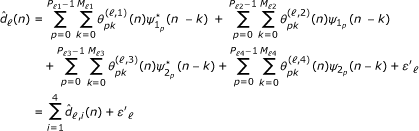(6)

where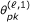and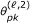denote the MP coefficients associated to the input signal and its conjugate, respectively;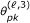and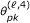are the coefficients associated to the crosstalk signal and its conjugate. The basis function of the corresponding MPs are defined by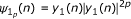and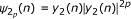. P i and M i are the polynomial order and memory depth of the branch i, respectively. The coefficient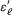represents the DC offsets that arise from the IQ modulators associated to the branches 1 and 2 of the transceiver.

It is straightforward to extend (6) to the more general case of M-antennas MIMO-PD by including, instead of the terms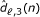and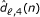, the corresponding 2(M - 1) terms characterizing the nonlinear crosstalk from the other branches.

According to the two-antenna PD to simplify the notation, we define the coefficient vector: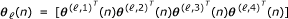, where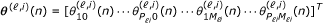(7)

with i = 1,...,4. Then, by defining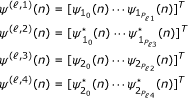(8)

with i = 1,... 4, the basis function vector can be written as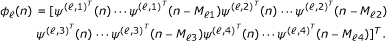(9)

The PD output of the branch can be written as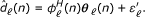(10)

To account for the DC offset from the LO an extra coefficient,, is added to the coefficient vector. Using an augmented coefficient vector, the PD output signal can be expressed as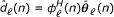(11)

where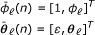(12)

where the augmented coefficient vector has dimensions 1 × (P 1M 1+ P 2M 2+ P 3M 3+ P 4M 4+ 1).

### B. MIMO predistorter identification schemes

To estimate the MP coefficients, θ (n) and to track the time-varying characteristics of the PA, adaptive estimation algorithms are considered. We propose two different algorithms: RLS and stochastic gradient algorithms. At the initialization, the PD is bypassed, and the PD coefficients are obtained by minimizing the error signal given by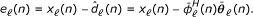(13)

Using the instantaneous squared error |e (n)|2 as an objective function, a stochastic gradient algorithm that updates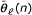is given by(14)

where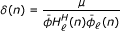and μ is a step size controlling the convergence speed and algorithm stability.

In the case of the recursive least squares algorithm, the deterministic objective function to be minimized is given by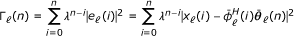(15)

where λ is the forgetting factor. The update process for the PD coefficients at each time instant n can be summarized as follows: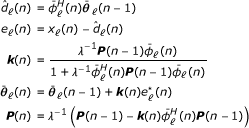(16)

where k(n) is the gain vector, and P(n) is the inverse correlation matrix.

In the RLS algorithm, the coefficient vector θ is initialized by θ (0) = [0, 1,... 0], and the inverse correlation matrix is initialized to P(0) = α I where I is a (P 1M 1+ P 2M 2+ P 3M 3+ P 4M 4+ 1) × (P 1M 1+ P 2M 2+ P 3M 3+ P 4M 4+ 1) identity matrix, and α is a large constant.

For comparison, we discuss an extension of the least squares (LS) algorithm of  that also includes IQ imbalance distortion at each MIMO-PD branch, in addition to nonlinear crosstalk. Also to maintain simple notation, we discuss the two-antenna predistorter. The estimated input signal for this case can be expressed as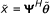(17)

where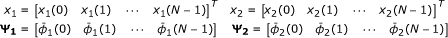(18)

Then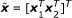is a (2N × 1) vector representing the N samples of the desired PD output of each branch, Ψ = [Ψ1Ψ2] is an (L1 + L2 + 1) × 2N matrix formed by the basis function defined by (9) and (12) (augmented basis function that includes an unitary term), and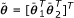is an (L1 + L2 + 1) × 1 vector formed by the MIMO-PD coefficients (including DC compensation coefficient). The coefficients vector size is defined as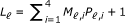with = 1,2. The LS solution for (17) is given by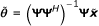(19)

In this estimator, the measurement noise affects the data matrix, Ψ while in the ordinary LS solution, the measurement noise lies in the observation vector,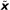. In this case, the estimator defined by Equation (19) is called Data Least Squares .

The performance and characteristics of this extension of the MIMO-PD of  are studied and compared with our proposal in Section 4.

## 4. Implementation Aspects of the MIMO Predistorter

The implementation of predistortion techniques involves two steps: PD coefficient estimation and predistortion using the estimated coefficients.

### A. Predistorter coefficients estimation

The dimensions of MP models, i.e., memory depth and polynomial order, need to be carefully chosen. Using a large polynomial order allows for coping with strong nonlinear responses which leads an improvement in the linearization capabilities of the MIMO-PD. On the other hand, overmodeling could deteriorate the numerical stability of the PD identification algorithms  and could make the estimation algorithms more sensitive to the measurement noise . Large polynomial order also decreases the interval of successful compensation (that reduces the PA dynamic range) . We study the trade-off between implementation complexity, linearization capabilities, robustness against noise, and algorithm stability by simulations in this section.

1) Estimation algorithms: The complexity of the estimation technique is directly related to the size of the coefficient vector. For example, the two-antenna PD proposed is formed by two independent PD blocks composed of four branches, each formed by a MP. The length of the coefficient vector of each block is given by the sum of the coefficients length of each branch: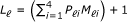. In case of LS implementation, its complexity is proportional to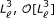. For the RLS algorithm, the complexity is reduced to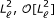. On the other hand, the complexity of stochastic gradient algorithms is proportional to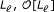. However, if the PA characteristic is highly nonlinear, it results in a poorly conditioned covariance matrix. This ill-conditioned covariance is reflected in slow convergence, when this kind of stochastic gradient algorithms is employed.

In order to reduce the implementation complexity of the RLS algorithm, the widely linear-RLS (WL-RLS)  algorithm can be evaluated in a future research. It is an interesting approach to reduce the implementation complexity of the RLS version of the MIMO-PD, obtaining similar convergence speed and robustness. This algorithm has an implementation complexity proportional to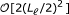, which is computationally more economical than the conventional RLS.

Note that the identification algorithms are not executed for every sample. It is done periodically depending on the variation of the PA parameters due to thermal effects (which usually vary slowly with the time). The PD parameter identification step is not a big consumer of computational resources because it is carried out only periodically. However, it should be kept in mind that when using LS algorithm, a large portion of memory is required to store the block of samples. In addition to the large complexity associated to the inversion of a huge matrix, as required by the LS implementation, memory requirements are another point that motivates the use of an RLS algorithm.

### B. MIMO-PD implementation

A MP with order P i and memory depth M i can be expressed as a parallel of M i MLPs of order P i .

Our implementation employs the input signal and its conjugate. Moreover, the branches associated to the signal and its conjugate share the same basis function, i.e.,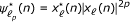. For this reason, its implementation does not require extra multiplications (only a conjugation operation).

The implementation of each MLP (considering the input signal x (n) and its conjugate) requires max{P i } complex-valued products to implement the basis function, P i + P i+1complex-valued products to weight the basis function with the polynomial coefficients, P i+1conjugations, and P i + P i+1additions. An extra addition is required to compensate the DC offset.

All PD block of the structure proposed ( = 1,..., M) employs the same basis functions, and so the complete PD requires the implementation of only one basis function (the one which have the largest polynomial order). Each branch i of the path composes the MIMO-PD, is formed by a MP implemented with M i MLPs. For this reason, to build a MP branch, the operations required to implement a MLP needs to be executed M i times with i = 1,..., 2 + 2(M - 1) and = 1,..., M. The block diagram of a 2 × 2 MIMO-PD is depicted in Figure 4a. The structure of one branch of the MIMO-PD is illustrated in Figure 4b. In Figure 4c, the implementation of a MLP is detailed.

Table 1 summarizes the operations required to implement the proposed MIMO-PD. For comparison, we also include the required operations to implement a conventional PD, CPD-1 assuming no coupling and an ideal IQ modulator, and a conventional PD, CPD-2 assuming nonlinear crosstalk compensation but not IQ imbalance reduction .

## 5. Performance Evaluation

In this section, we evaluate the performance of the proposed linearization techniques. First, we discuss the figures of merit evaluated and then the complete simulation setup is described and discussed.

### A. Figures of merit

The nonlinear effects introduced by the distortions considered (the nonlinear PA, IQ imbalances, and crosstalk), create in-band and out-of-band distortions. Two figures of merit are considered to evaluate the performance of the proposed linearization technique: the EVM (which quantifies the in-band distortion and is directly related to the BER), and the ACPR (which is a measure of the effects of the out-of-band distortion on adjacent channels).

#### EVM

In most of the standards, EVM is adopted to quantify the amount of in-band distortion that occurs at the transmitter side. EVM is expressed as the difference, e (k), between the original constellation points, X (k), and the recovered signal affected by system imperfections at the k th subcarrier of the th PA. The EVM for an OFDM system formed by N subcarriers is given by the average of EVM over active subcarriers: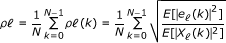(20)

where E[·]denotes the expectation operator, E[|X (k)|2] is the desired signal energy per symbol, ρ (k) is the EVM at subcarrier k, and e (k) is the error signal defined as e (k) = X (k) - Y (k), where Y (k) is the received frequency-domain signal after down-conversion.

In order to get the constellation after the PA and evaluate the degradation in terms of the EVM, the output signal is down-converted and demodulated via FFT. Since we are interested in the degradation of the transmitted signal, we assume that down-conversion and FFT demodulation processes, performed to demodulate the transmitted baseband signal, are carried by employing an ideal receiver.

#### ACPR

The out-of-band distortion is directly related to the PA operation point. The out-of-band emission increases when the PA is driven into its nonlinear operation region. This is also the region that allows high power efficiency. The ACPR is employed to characterize the spectral regrowth and is defined as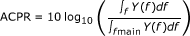(21)

where Y(f ) is the power spectral distribution at the output of the linearized PA. fad and fmain define the frequency bands of the adjacent and the main channels, respectively.

For example, mobile WiMAX standard defines an spectral mask that should be fulfilled. The PA requires to be operated as close as possible to the maximum efficiency point that met the ACPR and spectrum mask requirements. If these requirements cannot be met, then the PA operation point must be moved, where the mask and the ACPR are fulfilled reducing the PA efficiency. The adjacent channel frequency varies with the system application. In this study the ACPR is evaluated in an adjacent band (frequency offset) shifted 7 MHz from the main band.

### B. Simulations

The performance is validated with a two-antenna MIMO-OFDM transmitter, 16-QAM modulation on N = 1,024 subcarriers, and a bandwidth of 20 MHz. An oversampling factor of R = 8 with an interpolation filter based on root-raised cosine pulse shape with a roll-off factor of 20% has been employed. The coupling between the twobranch transmitter (after PA) is assumed to be frequency dependent and modeled by a FIR filter with impulse response c21 = ρ2[1, 0.2] (coupling from branch 2 to branch 1) and c12 = ρ1[1, 0.15] (coupling from branch 1 to branch 2) where ρ is a coupling factor employed to define the crosstalk level.

The amplitude and phase imbalance are β1 = 5% and ϑ1 = 5°; and β2 = 3% and ϑ1 = 3°, respectively. The frequency-dependent imbalance are modeled by FIR filters with the following impulse response: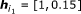and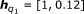for the branch 1, and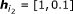and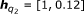. The DC offset are |ε1| = 0.1 and |ε2| = 0.07 with signal power normalized to 1.

The proposed MIMO-PD is evaluated for two different PA models:

Power amplifier 1 (PA-1): Class A amplifier. The PA is modeled by a Wiener model where static nonlinearity corresponds to a solid-state power amplifier (SSPA) which is modeled by the Saleh model , i.e.,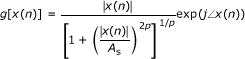(22)

where the parameter p = 1.2 adjusts the smoothness of the transition from the linear region to the saturation region, and A s is the amplifier input saturation. The PA operation point is set 2 dB from the 1-dB compression point. PA memory effects are modeled with a FIR filter with coefficients h p = [1, 0.25, 0.1].

Power amplifier 2 (PA-2): Class AB amplifier. The PA is modeled by a Wiener model taken from . The static nonlinearity is modeled with a polynomial and can be expressed as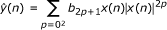(23)

where the complex-valued coefficients are b1 = 14.97+0.0519j, b3 = - 23.0954+4.968j, and b5 = 21.3936 + 0.4305j. The linear filter is given by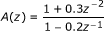(24)

We assume identical polynomial order and memory depth for each PD branch, i.e., M, i= 6 and P, i= P = 4 for i = 1,..., 4 and = 1, 2. However, owing to the difference between the power of the useful signal and the power of the conjugate component, different polynomial order and memory depth can be considered in each branch to optimize the implementation complexity .

For PD coefficient estimation, we evaluate the RLS, the stochastic gradient, and the LS algorithms. For comparison, we also evaluate the performance of a conventional PD (CPD-1) that compensates PA memory effects but neglects IQ imbalance and crosstalk, i.e., it is assumed that each branch of the MIMO transceiver is decoupled. We also implemented a conventional predistorter, denoted CPD-2, that compensates PA effects and crosstalk effects neglecting IQ imbalances .

#### Learning curves

Figure 5a, b shows the learning curves of the MIMO-PD for the stochastic gradient and RLS algorithms using the SSPA and class AB models, respectively. We see that for the PA-1, the RLS algorithm only requires five OFDM symbols to reach the MSE steady state. The stochastic gradient algorithm, which has a reduced implementation complexity, requires ten OFDM symbols to reach the convergence. When conventional PDs (CPD-1, CPD-2) are used, the MSE floor is increased, reflecting this performance in EVM and ACPR. For the class AB PA, which presents a moderate nonlinearity at low and high amplitudes, the stochastic gradient algorithm is seriously affected by a poorly conditioned covariance matrix and cannot reach the convergence even for a large training sequence (30 OFDM symbols). These results lead us to conclude that the RLS algorithm is the best option to the estimation of PD coefficients.

#### Linearization capabilities

Figure 6 shows the AM-AM and AM-PM curves before and after linearization for PA-1. Identical results are observed for our MIMO-PD estimated using stochastic gradient, RLS and LS algorithms. These curves also show that conventional PDs are unable to linearize the MIMO transmitter. Residual memory effects are observed in AM-AM and AM-PM figures. These results can also be observed in the constellation map at the linearized PA output depicted in Figure 7, where the impact of the crosstalk and IQ imbalance can be observed. Figures 6 and 7 had been obtained including a DC offset, |ε1| = |ε2| = 0.1.

Spectral regrowth curves are shown in Figure 8 for a measurement noise of 40 dB and crosstalk coupling ρ = -20 dB, using a class A PA (PA-1). In an identical scenario, the in-band distortion was evaluated showing EVM values around -30 dB, when using LS, RLS, and SG identification algorithms, and for a practical SNR = 40 dB. Conventional PDs present a poor performance when the in-band distortion is evaluated, giving unsuitable levels of EVM. ACPR and EVM results are summarized in Table 2 with crosstalk coupling of ρ = -20 dB, ρ = -30 dB and without coupling. Effects of polynomial order. The effects of the PD polynomial order over the MIMO-PD performance were also evaluated. MIMO-PDs with orders 3, 4, 5 (only odd-orders) and a full odd and even order (P = 3) are considered. ACPR curves, depicted in Figure 9, indicate that when the polynomial order is increased, the performance of the MIMO-PD is diminished for moderate SNR. For infinite SNR (without measurement noise), the increment of polynomial order has no noticeable effect in the system performance. The performance of the different implementations in terms of EVM and ACPR is summarized in Table 3. These results demonstrate that overmodeling degrades the PD performance or at least increases the implementation complexity without any extra advantages in terms of performance.

#### Effects of measurement noise

We evaluate the MIMO-PD performance against measurement noise for both PAs. ACPR and EVM curves versus measurement noise are illustrated in Figure 10a,b, respectively, using PA-1. Figure 10c,d, and 10b shows ACPR and EVM results for PA-2, respectively. These curves indicate that reasonable values of ACPR and EVM are obtained for SNR larger than 35 dB. This SNR level can be easily obtained in a practical scenario. These results show that PD using the largest polynomial order give the worst performance in terms of EVM and ACPR in the low SNR region. At high SNR, MIMO-PDs using different polynomial orders reach similar results.

## 6. Conclusions and Future Work

We have presented a MIMO-PD that combines PA response linearization, IQ imbalance, and crosstalk compensation. Our PD shows an improved performance compared with conventional isolated PD structures. EVM values around -30 dB, and ACPR nearer to 50 dB are obtained with the proposed MIMO-PD in a scenario that includes impairments at the upconversion block modulator and crosstalk between the different branches. Conventional PDs are unable to operate in this scenario, giving EVM and ACPR values that fail to comply with the specifications of the majority of the wireless standards. The proposed technique shows a moderate implementation complexity and also includes tracking capabilities to follow PA parameter variations. Simulation results show that the MIMO-PD works appropriately in a realistic measurement noise scenario.

Through a future study, the WL-RLS algorithm can be evaluated. The WL-RLS approach is computational more economical than the conventional RLS obtaining similar convergence speed and robustness. Reduced complexity techniques and robustness are interesting issues which need to be addressed in future research.

## Abbreviations

ACPR:

adjacent channel power ratio

BER:

bit error rate

DAC:

digital-analog converters

EVM:

error vector magnitude

IQ:

LO:

local oscillator

MIMO:

multiple input multiple output

MLP:

memoryless polynomials

MP:

memory polynomial

PA:

power amplifier

PD:

predistortion.

## References

1. Eun C, Powers EJ: A new Volterra predistorter based on the indirect learning architecture. IEEE Trans Signal Process 1997,45(1):223-227. 10.1109/78.552219

2. Ding L, Zhou GT, Morgan DR, Ma Z, Kenney JS, Kim J, Giardina CR: A robust digital baseband predistorter constructed using memory polynomials. IEEE Trans Commun 2004,52(1):159-165. 10.1109/TCOMM.2003.822188

3. Gilabert P, Montoro G, Bertran E: On the Wiener and Hammerstein models for power amplifier predistortion. Proceedings of the Asia-Pacific Microwave Conference, APMC 2005 2005.

4. Gregorio F, Werner S, Cousseau J, Laakso T: Receiver cancellation technique for nonlinear power amplifier distortion in SDMA-OFDM systems. IEEE Trans Veh Technol 2007,56(5 Part I):2499-2516.

5. Cavers J: The effect of quadrature modulator and demodulator errors on adaptive digital predistorters for amplifier linearization. IEEE Trans Veh Technol 1997,46(2):456-466. 10.1109/25.580784

6. Cavers J, Liao M: Adaptive compensation for imbalance and offset losses in direct conversion transceivers. IEEE Trans Veh Technol 1993,42(4):581-588. 10.1109/25.260752

7. Cavers J: New methods for adaptation of quadrature modulators and demodulators in amplifier linearization circuits. IEEE Trans Veh Technol 1997,46(3):707-716. 10.1109/25.618196

8. Ding L, Ma Z, Morgan D, Zierdt M, Tong Zhou G: Compensation of frequency-dependent gain/phase imbalance in predistortion linearization systems. IEEE Trans Circuits Syst I 2008,55(1):390-397.

9. Anttila L, Handel P, Valkama M: Joint mitigation of power amplifier and IQ modulator impairments in broadband direct-conversion transmitters. IEEE Trans Microw Theory Tech 2010,58(4):730-739.

10. Zareian H, Vakili VT: New adaptive method for IQ imbalance compensation of quadrature modulators in pre-distortion systems. EURASIP J Adv Signal Process 2009, 10. Article ID 181285

11. Kim Y-D, Jeong E-R, Lee YH: Adaptive compensation for power amplifier nonlinearity in the presence of quadrature modulation/demodulation errors. IEEE Trans Signal Process 2007,55(9):4717-4721.

12. Palaskas Y, Ravi A, Pellerano S, Carlton B, Elmala M, Bishop R, Banerjee G, Nicholls R, Ling S, Dinur N, Taylor S, Soumyanath K: A 5-GHz 108-Mb/s 2×2 MIMO transceiver RFIC with fully integrated 20.5-dBm power amplifiers in 90-nm CMOS. IEEE J Solid-State Circuits 2006,41(12):2746-2756.

13. Bassam S, Helaoui M, Ghannouchi F: Crossover digital predistorter for the compensation of crosstalk and nonlinearity in MIMO transmitters, IEEE Trans Microw. Theory Tech 2009,57(5):1119-1128.

14. Morgan D, Ma Z, Kim J, Zierdt M, Pastalan J: A generalized memory polynomial model for digital predistortion of RF power amplifiers. IEEE Trans Signal Process 2006,54(10):3852-3860.

15. Morgan D, Ma Z, Ding L: Reducing measurement noise effects in digital predistortion of RF power amplifiers. Proceedings of the IEEE International Conference on Communications, ICC 2003, 4: 2436-2439.

16. Mak P-I, Seng-Pan U, Martins R: Transceiver architecture selection: review, state-of-the-art survey and case study. IEEE Circuits Syst Mag 2007,7(2):6-25.

17. Masse C, Luu Q: A 2.4 GHz WiMAX direct conversion transmitter, Analog Devices. AN-826 Application note 2007, 1-16.

18. Tandur D, Moonen M: Joint compensation of OFDM frequency-selective transmitter and receiver IQ imbalance. EURASIP J Wireless Commun Networking 2007, 10. Article ID 68563

19. Liu CL: Impacts of IQ imbalance on QPSK-OFDM-QAM detection. IEEE Trans Consum Electron 1998,44(3):984-989. 10.1109/30.713223

20. Li M, Hoover L, Gard KG, Steer MB: Behavioral modeling and impact analysis of physical impairments in quadrature modulators. IET, Trans Microwaves Antennas Propag 2010,4(12):2144-2154. 10.1049/iet-map.2009.0278

21. Raich R, Qian H, Zhou GT: Orthogonal polynomials for power amplifier modeling and predistorter design. IEEE Trans Veh Technol 2004,53(5):1468-1479. 10.1109/TVT.2004.832415

22. Golub G, Loan CFV: Matrix Computations. The Johns Hopkins University Press, Baltimore; 1993.

23. DeGroat RD, Dowling EM: The data least squares problem and channel equalization. IEEE Trans Signal Process 1993,41(1):407-411. 10.1109/TSP.1993.193165

24. Messaoudi N, Fares M-C, Boumaiza S, Wood J: Complexity reduced odd-order memory polynomial pre-distorter for 400-watt multi-carrier Doherty amplifier linearization. 2008 IEEE MTT-S International Microwave Symposium Digest 2008, 419-422.

25. Tsimbinos J: Identification and compensation of nonlinear distortion. Ph.D thesis, Institute for Telecommunications Research, School of Electronic Engineering, University of South Australia 1995.

26. Douglas S: Widely-linear recursive least-squares algorithm for adaptive beamforming. IEEE International Conference on Acoustics, Speech and Signal Processing 2009, 2041-2044.

27. Saleh AAM: Frequency-independent and frequency-dependent nonlinear models of TWT amplifiers. IEEE Trans Commun 1981,29(11):1715-1720. 10.1109/TCOM.1981.1094911

28. Ding L: Digital predistortion of power amplifiers for wireless applications. Ph.D thesis, School of Electrical and Computer Engineering, Georgia Institute of Technology 2004.

## Acknowledgements

This work was partially supported by the Academy of Finland, Smart Radios (SMARAD) Center of Excellence, Agencia Nacional de Promoción Científica y Tecnológica PICT 2008-00104 and PICT 2008-0182, and Universidad Nacional del Sur, Argentina, Project 24/K044.

## Author information

Authors

### Corresponding author

Correspondence to Fernando Gregorio.

### Competing interests

The authors declare that they have no competing interests.

## Authors’ original submitted files for images

Below are the links to the authors’ original submitted files for images.

## Rights and permissions

Open Access This article is distributed under the terms of the Creative Commons Attribution 2.0 International License (https://creativecommons.org/licenses/by/2.0), which permits unrestricted use, distribution, and reproduction in any medium, provided the original work is properly cited.

Reprints and Permissions

Gregorio, F., Cousseau, J., Werner, S. et al. Power amplifier linearization technique with IQ imbalance and crosstalk compensation for broadband MIMO-OFDM transmitters. EURASIP J. Adv. Signal Process. 2011, 19 (2011). https://doi.org/10.1186/1687-6180-2011-19

• Accepted:

• Published:

• DOI: https://doi.org/10.1186/1687-6180-2011-19

### Keywords

• RF impairments
• MIMO
• predistortion
• nonlinear crosstalk